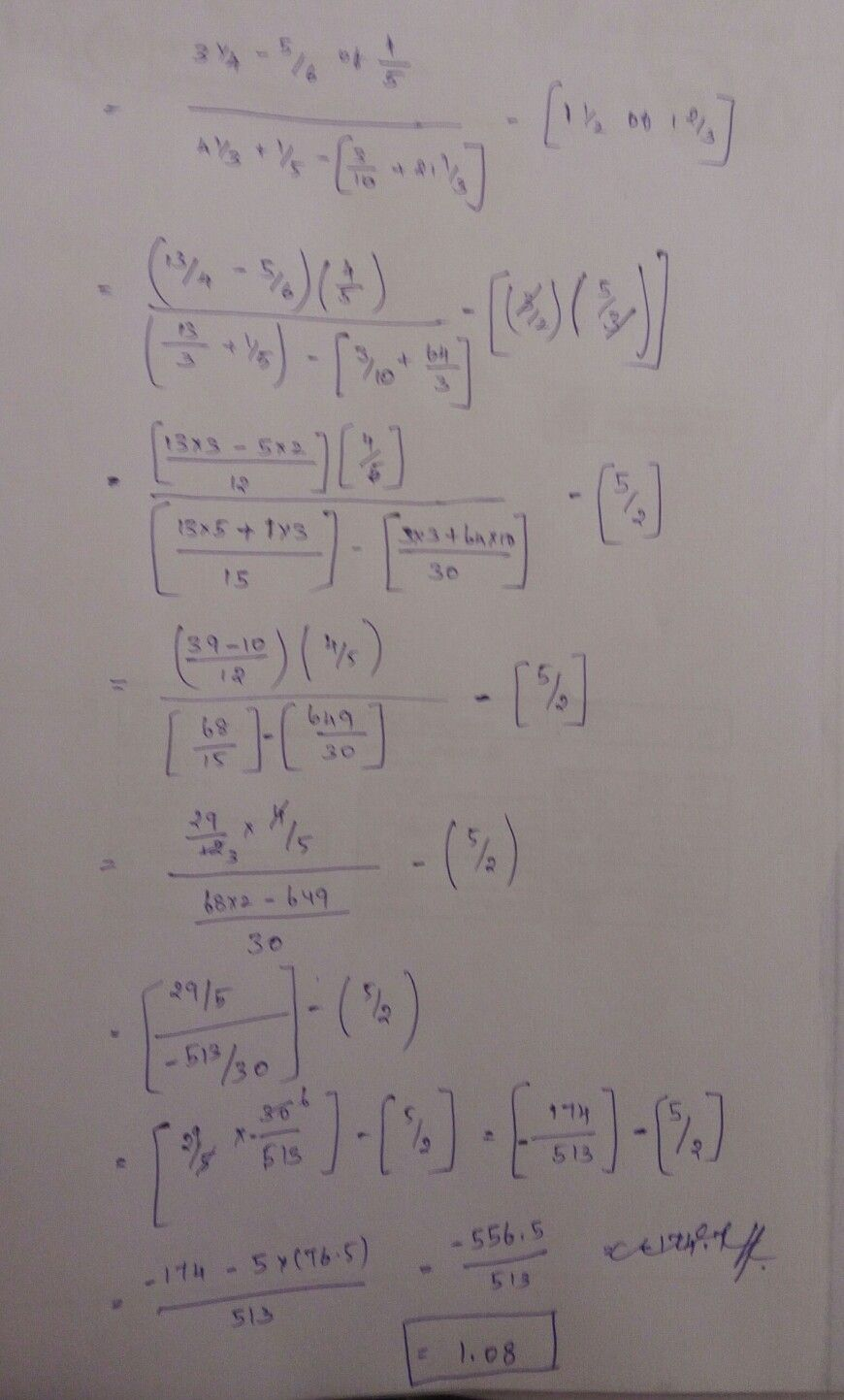Symbol
Problem$\dfrac {3\dfrac {1} {4}-\dfrac {5} {6}x\dfrac {4} {5}} {4\dfrac {1} {3}+\dfrac {1} {5}\left(\dfrac {3} {10}+21\dfrac {1} {3}\right)}-\left(1\dfrac {1} {2}$ $\bar{5}$ $1$ $-$ $1\dfrac {2} {3}\right)$
10th-13th grade
Other
SolutionQanda teacher - Ragavi
am doing it dearThank you dear.. if you have any queries get back to me dear